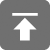# 工学1号馆

home

## Project Euler 6–Sum square difference

Wu Yudong    January 14, 2017     欧拉计划   448

The sum of the squares of the first ten natural numbers is,
12 + 22 + … + 102 = 385
The square of the sum of the first ten natural numbers is,
(1 + 2 + … + 10)2 = 552 = 3025
Hence the difference between the sum of the squares of the first ten natural numbers and the square of the sum is 3025 − 385 = 2640.

Find the difference between the sum of the squares of the first one hundred natural numbers and the square of the sum.

12 + 22 + … + 102 = 385

(1 + 2 + … + 10)2 = 552 = 3025

#include <stdio.h>
#include <string.h>
#include <ctype.h>
#include <math.h>

#define N 100

int powplus(int n, int k)
{
int s = 1;
while (k--) {
s *= n;
}
return s;
}

int sum1(int n)
{
return powplus((n + 1) * n / 2, 2);
}

int sum2(int n)
{
return (n * (n + 1) * (2 * n + 1)) / 6;
}

void solve()
{
printf("%d\n", sum1(N));
printf("%d\n", sum2(N));
printf("%d\n", sum1(N) - sum2(N));
}

int main()
{
solve();
return 0;
}